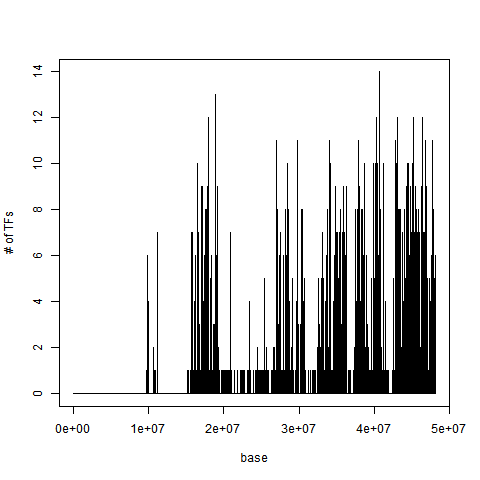# 1 Introduction

Transcription factors (TFs) are proteins that bind the DNA in specific regions and regulate gene expression. The regolation of the gene expression is often controlled by many TFs interacting with each other. Recent high throughput methods like chromatin immunoprecipitation followed by sequencing (ChIP-seq)1 provide a large number of data regarding TF binding regions, which are available in public repositories such as ENCODE2 or Roadmap Epigenomics3.
Starting from a dataset containing the genomic positions of TF binding regions, the TFHAZ package allows finding trascription factor high accumulation DNA zones, i.e., regions along the genome where there is a high presence of different transcription factors. In addition, some functions are provided in order to analyze and compare results obtained with different input parameters.

# 2 Dataset

Transcription factor dense DNA zones are found from a GRanges object that contains genomic regions of transcription factors at the ranges side and the name of the transcription factors at the metadata side. As in every object of GRanges class, the genomic coordinates are located on the left-hand side. The first column of the ranges side contains the chromosome of each region; the second column contains the genomic coordinates of each region (starting and ending position of the transcription factor binding region, considering a 1-based inclusive coordinate system); the third column contains the strand of each region (“+”, “-”, or “*” if unknown).
The dataset we consider (called Ishikawa) is obtained from computation of ENCODE ChIP-Seq data of the localization of transcription factor binding regions for the Ishikawa cell line. The data have been processed and extracted with GMQL (GenoMetric Query Language http://www.bioinformatics.deib.polimi.it/GMQL/)456. The Ishikawa dataset contains 283,009 ranges of 16 different transcription factors.

``````# load and visualize the dataset:
data("Ishikawa")
dim(as.data.frame(Ishikawa))``````
``##  283009      6``
``head(Ishikawa)``
``````## GRanges object with 6 ranges and 1 metadata column:
##       seqnames           ranges strand |         TF
##          <Rle>        <IRanges>  <Rle> |   <factor>
##        chr1 [840014, 840266]      * | NFIC-human
##        chr1 [841252, 841697]      * | NFIC-human
##        chr1 [856253, 856932]      * | NFIC-human
##        chr1 [858967, 859537]      * | NFIC-human
##        chr1 [859558, 860319]      * | NFIC-human
##        chr1 [867077, 867783]      * | NFIC-human
##   -------
##   seqinfo: 24 sequences from an unspecified genome; no seqlengths``````

# 3 Trascription factor accumulation

The first step in finding transcription factor dense DNA zones is to count the accumulation of TFs for each chromosome base. The function accumulation in the TFHAZ package creates a vector in which, for each chromosome base, the accumulation of the TFs present in the input dataset is calculated. We considered three types of accumulation: TF accumulation, region accumulation and base accumulation.

TF accumulation: for each base, it is the number of different TFs present in the neighborhood of the considered base. The neighborhood is defined by a window with half-width w centered on the considered base.

Region accumulation: for each base, it is the number of regions containing TFs in the neighborhood of the considered base. If in the neighborhood of a base there are two input binding regions of the same TF, the accumulation value in that base is equal to 2 (differently from the TF accumulation, whose value in the same case is equal to 1).

Base accumulation: for each base, it is the total number of bases belonging to input regions containing TFs in the neighborhood of the considered base.

With w=0, a single base approach is applied (no base neighborhood is considered). In this case, if in the input dataset overlapping regions for the same TF and chromosome do not exist, the results of TF, region and base accumulation are equal.

The function accumulation takes in input:

• a GRanges object containing coordinates of TF binding regions and their TF name;
• a string with the name of the accumulation type: “TF”, “region”, “base”;
• a string with the name of the chromosome (e.g., “chr1”);
• an integer, half-width of the window that defines the neighborhood of each base.

The result of the accumulation function is a list containing:

• accvector: a sparse vector containing the accumulation for each base of the selected chromosome;
• acctype: a string with the accumulation type used;
• chr: a string with the chromosome name associated with the accumulation vector;
• w: an integer with the half-width of the window used to calculate the accumulation vector.

The accumulation vector obtained can be plotted using the function plot_accumulation.
This function takes in input the output of the accumulation function and saves the plot in a .png file.

``````# calculate TF accumulation for the chromosome 21 for w=0
TF_acc_21_w_0 <- accumulation(Ishikawa, "TF", "chr21", 0)``````
``````# plot the accumulation vector
plot_accumulation(TF_acc_21_w_0)``````Figure 1: Figure 1: Plot of the TF accumulation vector for the chromosome 21, obtained for w=0

As we can see in Figure 1, in this example considering no base neighborhood (w=0), the maximum value of accumulation found is 14. It means that in the bases with that accumulation value there are overlapping binding regions of 14 out of the 16 transcription factors present in the dataset.

# 4 Transcription factor dense DNA zones

Once the accumulation for each chromosome base is calculated, we can find transcription factor dense zones with the dense_zones function. For each accumulation threshold value defined, the function finds transcription factor dense DNA zones (regions). Starting from the accumulation vector calculated with the accumulation function, each dense zone is formed by contiguous bases with accumulation equal or higher than the threshold. Threshold values are selected by setting the threshold_step parameter.
For each accumulation threshold value, a “.bed” file with the chromosome and genomic coordinates of the dense zones found is created (Figure 2). The function finds also the number of dense zones, the number of total bases belonging to the dense zones, the m